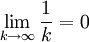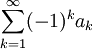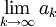Previous: An Alternating Series Test Concept Map

Next: Alternating Series Test Videos

## Example

We now return to the example we presented at the beginning of the lesson:We wish to determine whether it is convergent using the alternating series test.

## Complete Solution

### Step 1: Check to see if the alternating series test can be applied

We see that the terms ak = 1/k satisfy: ak+1 < ak. Moreover, the terms in the sequence alternate between positive and negative.

Therefore the alternating series test can be applied.

### Step 2: Apply the Alternating Series TestThe series is therefore convergent.

## Explanation of Each Step

### Step (1)

Given a general alternating series,we need only two criteria to apply the alternating series test for a given infinite series:

1. terms in the series must alternate between positive and negative
2. the terms in the sequence must be decreasing (in other words, ak < ak-1)

Our series meets these two criteria.

### Step (2)

The alternating series test on the general alternating seriesonly requires that we evaluateIf the limit is zero, the series is convergent. In this case, the limit is zero, so our sequence is convergent.

Previous: An Alternating Series Test Concept Map

Next: Alternating Series Test Videos